# Basic drawing in PyCairo

In this part of the PyCairo tutorial, we draw some basic primitives. We use fill and stroke operations, dashes, line caps, and line joins.

## Lines

Lines are very basic vector objects. To draw a line, we use two method calls. The starting point is specified with the `move_to()` call. The ending point of a line is specified with the `line_to()` call.

lines.py
```#!/usr/bin/python

'''
ZetCode PyCairo tutorial

In this program, we connect all mouse
clicks with a line.

Author: Jan Bodnar
Website: zetcode.com
Last edited: April 2016
'''

from gi.repository import Gtk, Gdk
import cairo

class MouseButtons:

LEFT_BUTTON = 1
RIGHT_BUTTON = 3

class Example(Gtk.Window):

def __init__(self):
super(Example, self).__init__()

self.init_ui()

def init_ui(self):

self.darea = Gtk.DrawingArea()
self.darea.connect("draw", self.on_draw)
self.darea.set_events(Gdk.EventMask.BUTTON_PRESS_MASK)
self.add(self.darea)

self.coords = []

self.darea.connect("button-press-event", self.on_button_press)

self.set_title("Lines")
self.resize(300, 200)
self.set_position(Gtk.WindowPosition.CENTER)
self.connect("delete-event", Gtk.main_quit)
self.show_all()

def on_draw(self, wid, cr):

cr.set_source_rgb(0, 0, 0)
cr.set_line_width(0.5)

for i in self.coords:
for j in self.coords:

cr.move_to(i, i)
cr.line_to(j, j)
cr.stroke()

del self.coords[:]

def on_button_press(self, w, e):

if e.type == Gdk.EventType.BUTTON_PRESS \
and e.button == MouseButtons.LEFT_BUTTON:

self.coords.append([e.x, e.y])

if e.type == Gdk.EventType.BUTTON_PRESS \
and e.button == MouseButtons.RIGHT_BUTTON:

self.darea.queue_draw()

def main():

app = Example()
Gtk.main()

if __name__ == "__main__":
main()
```

In our example, we click randomly on the window with a left mouse button. Each click is stored in a list. When we right click on the window, all points are connected with every other point in the list. Additional right click clears the window.

```class MouseButtons:

LEFT_BUTTON = 1
RIGHT_BUTTON = 3
```

The GTK documentation simply states that the left mouse button has number 1, right mouse button number 3. We create a custom class to have some identifiers for the mouse buttons.

```self.darea.set_events(Gdk.EventMask.BUTTON_PRESS_MASK)
```

Some events are not enabled by default; mouse press events are among them. Therefore, we need to enable mouse press events with the `set_event()` method.

```self.darea.connect("button-press-event", self.on_button_press)
```

In this code example, we react to mouse press events.

```cr.set_source_rgb(0, 0, 0)
cr.set_line_width(0.5)
```

The lines are drawn in black ink and are 0.5 points wide.

```for i in self.coords:
for j in self.coords:

cr.move_to(i, i)
cr.line_to(j, j)
cr.stroke()
```

We connect every point from the list to every other point. The `stroke()` call draws the lines.

```del self.coords[:]
```

In the end, all the coordinates are deleted. We can now create another object.

```def on_button_press(self, w, e):

if e.type == Gdk.EventType.BUTTON_PRESS \
and e.button == MouseButtons.LEFT_BUTTON:

self.coords.append([e.x, e.y])
...
```

If we press a left mouse button, we add its x and y coordinates to the `self.coords` list.

```if e.type == Gdk.EventType.BUTTON_PRESS \
and e.button == MouseButtons.RIGHT_BUTTON:

self.darea.queue_draw()
```

In case of a right mouse button press, we call the `queue_draw()` method which redraws the drawing area. All the points are connected with lines.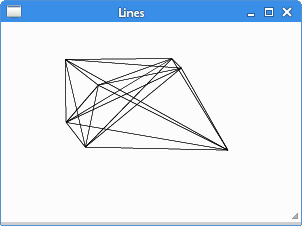Figure: Lines

## Fill and stroke

The stroke operation draws the outlines of shapes and the fill operation fills the insides of shapes.

fillstroke.py
```#!/usr/bin/python

'''
ZetCode PyCairo tutorial

This code example draws a circle
using the PyCairo library.

Author: Jan Bodnar
Website: zetcode.com
Last edited: April 2016
'''

from gi.repository import Gtk
import cairo
import math

class Example(Gtk.Window):

def __init__(self):
super(Example, self).__init__()

self.init_ui()

def init_ui(self):

darea = Gtk.DrawingArea()
darea.connect("draw", self.on_draw)
self.add(darea)

self.set_title("Fill & stroke")
self.resize(230, 150)
self.set_position(Gtk.WindowPosition.CENTER)
self.connect("delete-event", Gtk.main_quit)
self.show_all()

def on_draw(self, wid, cr):

cr.set_line_width(9)
cr.set_source_rgb(0.7, 0.2, 0.0)

w, h = self.get_size()

cr.translate(w/2, h/2)
cr.arc(0, 0, 50, 0, 2*math.pi)
cr.stroke_preserve()

cr.set_source_rgb(0.3, 0.4, 0.6)
cr.fill()

def main():

app = Example()
Gtk.main()

if __name__ == "__main__":
main()
```

In the example, we draw a circle and fill it with a solid color.

```import math
```

This module is needed for the `pi` constant which is used to draw a circle.

```cr.set_line_width(9)
cr.set_source_rgb(0.7, 0.2, 0.0)
```

We set a line width with the `set_line_width()` method. We set the source to some dark red color using the `set_source_rgb()` method.

```w, h = self.get_size()
```

Here we get the width and height of the window. We need these values to center the circle on the window.

```cr.translate(w/2, h/2)
cr.arc(0, 0, 50, 0, 2*math.pi)
cr.stroke_preserve()
```

With the `translate()` method, we move the drawing origin to the center of the window. We want our circle to be centered. The `arc()` method adds a new circular path to the Cairo drawing context. Finally, the `stroke_preserve()` method draws the outline of the circle. Unlike the `stroke()` method, it also preserves the shape for later drawing.

```cr.set_source_rgb(0.3, 0.4, 0.6)
cr.fill()
```

We change the color for drawing and fill the circle with a new color using the `fill()` method.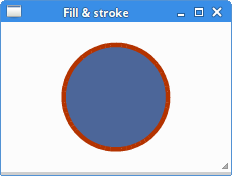Figure: Fill & stroke

## Pen dashes

Each line can be drawn with a different pen dash. A pen dash defines the style of the line. The dash pattern is specified by the `set_dash()` method. The pattern is set by the dash list which is a list of floating values. They set the on and off parts of the dash pattern. The dash is used by the `stroke()` method to create a line. If the number of dashes is 0, dashing is disabled. If the number of dashes is 1, a symmetric pattern is assumed with alternating on and off portions of the size specified by the single value in dashes.

```def on_draw(self, wid, cr):

cr.set_source_rgba(0, 0, 0, 1)
cr.set_line_width(2)

cr.set_dash([4.0, 21.0, 2.0])

cr.move_to(40, 30)
cr.line_to(250, 30)
cr.stroke()

cr.set_dash([14.0, 6.0])

cr.move_to(40, 50)
cr.line_to(250, 50)
cr.stroke()

cr.set_dash([1.0])

cr.move_to(40, 70)
cr.line_to(250, 70)
cr.stroke()
```

We draw three lines in three different pen dashes.

```cr.set_dash([4.0, 21.0, 2.0])
```

We have a pattern of three numbers. We have 4 points drawn, 21 not drawn, and 2 drawn, then 4 points not drawn, 21 points drawn. and 2 not drawn. This pattern takes turns until the end of the line.

```cr.set_dash([14.0, 6.0])
```

In this pattern, we have always 14 points drawn and 6 not drawn.

```cr.set_dash([1.0])
```

Here we create a pen dash of a symmetric pattern of alternating single on and off points.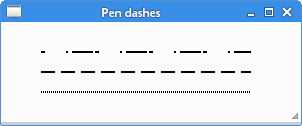Figure: Pen dashes

## Line caps

The line caps are end points of lines.

• cairo.LINE_CAP_BUTT
• cairo.LINE_CAP_ROUND
• cairo.LINE_CAP_SQUARE

There are three different line cap styles in Cairo.Figure: Square, round and butt caps

A line with a `cairo.LINE_CAP_SQUARE` cap has a different size than a line with a `cairo.LINE_CAP_BUTT` cap. If a line is x units wide, the line with a `cairo.LINE_CAP_SQUARE` cap will be exactly x units greater in size; x/2 units at the beginning and x/2 units at the end.

```def on_draw(self, wid, cr):

cr.set_source_rgba(0, 0, 0, 1)
cr.set_line_width(12)

cr.set_line_cap(cairo.LINE_CAP_BUTT)
cr.move_to(30, 50)
cr.line_to(150, 50)
cr.stroke()

cr.set_line_cap(cairo.LINE_CAP_ROUND)
cr.move_to(30, 90)
cr.line_to(150, 90)
cr.stroke()

cr.set_line_cap(cairo.LINE_CAP_SQUARE)
cr.move_to(30, 130)
cr.line_to(150, 130)
cr.stroke()

cr.set_line_width(1.5)

cr.move_to(30, 35)
cr.line_to(30, 145)
cr.stroke()

cr.move_to(150, 35)
cr.line_to(150, 145)
cr.stroke()

cr.move_to(155, 35)
cr.line_to(155, 145)
cr.stroke()
```

The example draws three lines with three different line caps. It will also graphically demonstrate the differences in size of the lines by drawing three additional thin vertical lines.

```cr.set_line_width(12)
```

Our lines will be 12 units wide. The default line width is 2.

```cr.set_line_cap(cairo.LINE_CAP_ROUND)
cr.move_to(30, 90)
cr.line_to(150, 90)
cr.stroke()
```

Here we draw a horizontal line with a `cairo.LINE_CAP_ROUND` cap.

```cr.set_line_width(1.5)

cr.move_to(30, 35)
cr.line_to(30, 145)
cr.stroke()
```

This is one of the three vertical lines used to demostrate the differences in size.Figure: Line caps

## Line joins

The lines can be joined using three different join styles.

• cairo.LINE_JOIN_MITER
• cairo.LINE_JOIN_BEVEL
• cairo.LINE_JOIN_ROUND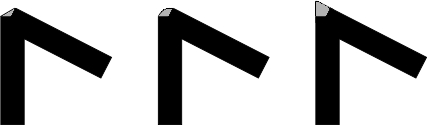Figure: Bevel, Round, Miter line joins
```def on_draw(self, wid, cr):

cr.set_line_width(14)

cr.rectangle(30, 30, 100, 100)
cr.set_line_join(cairo.LINE_JOIN_MITER)
cr.stroke()

cr.rectangle(160, 30, 100, 100)
cr.set_line_join(cairo.LINE_JOIN_BEVEL)
cr.stroke()

cr.rectangle(100, 160, 100, 100)
cr.set_line_join(cairo.LINE_JOIN_ROUND)
cr.stroke()
```

In this example, we draw three thick rectangles with various line joins.

```cr.set_line_width(14)
```

The lines are 14 units wide.

```cr.rectangle(30, 30, 100, 100)
cr.set_line_join(cairo.LINE_JOIN_MITER)
cr.stroke()
```

Here we draw a rectangle with `cairo.LINE_JOIN_MITER` join style.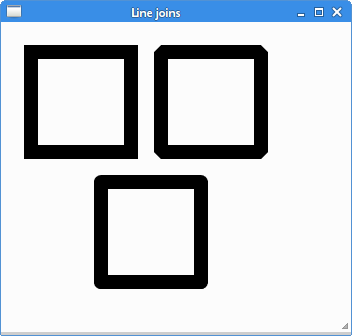Figure: Line joins

## Bézier curve

Bézier curves are curved lines defined by mathematical formulas. The mathematical method for drawing curves was created by Pierre Bézier in the late 1960's for the manufacturing of automobiles at Renault.

```curve_to(x1, y1, x2, y2, x3, y3)
```

The `curve_to()` method adds a cubic Bézier spline to the path. The parameters are the x and y coordinates of the first control point, x and y coordinates of the second control point, and the x and y coordinates of the end of the curve.

```def on_draw(self, wid, cr):

cr.move_to(20, 40)
cr.curve_to(320, 200, 330, 110, 450, 40)
cr.stroke()
```

In the example, a Bézier curve is drawn with the `curve_to()` method.Figure: Bézier curve

In this chapter of the PyCairo tutorial, we did some basic drawing.

ZetCode last modified April 19, 2016 © 2007 - 2019 Jan Bodnar Follow on Facebook